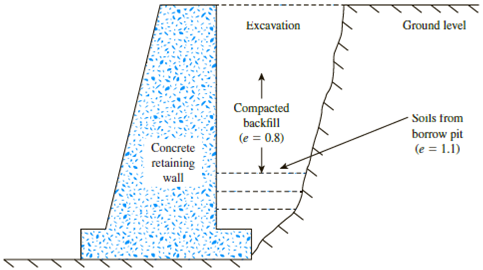Chapter 3, Problem 3.23PPrinciples of Geotechnical Enginee...

9th Edition
Braja M. Das + 1 other
ISBN: 9781305970939

Solutions

Chapter
SectionPrinciples of Geotechnical Enginee...

9th Edition
Braja M. Das + 1 other
ISBN: 9781305970939
Textbook Problem

Refer to Problem 3.22. Given that the borrow pit soil has a moisture content of 11% and Gs = 2.7, determinea. Moist unit weight of the borrow soilb. Degree of saturation of the borrow soilc. Moist unit weight of the compacted backfill3.22 Refer to Figure 3.14. After the construction of a concrete retaining wall, backfill material from a nearby borrow pit was brought into the excavation behind the wall and compacted to a final void ratio of 0.8. Given that the soil in the borrow pit has void ratio of 1.1, determine the volume of borrow material needed to construct 1 m3 of compacted backfill.Figure 3.14

(a)

To determine

Calculate the moist unit weight of the borrow soil.

Explanation

Given information:

The void ratio of compacted backfill (ebackfill) is 0.8.

The void ratio of borrow pit (eborrow) is 1.1.

The volume of compacted backfill (V) is 1m3.

The specific gravity of soil solids (Gs) is 2.7.

The moisture content of borrow pit soil (w) is 11%.

Calculation:

Consider the unit weight of water (γw) is 9.81kN/m3.

Calculate the moist unit weight of the borrow soil (γborrow) using the relation.

γborrow=(1+w)Gsγw1+eborrow

Substitute 11% for w, 2

(b)

To determine

Calculate the degree of saturation of the borrow soil.

(c)

To determine

Calculate the moist unit weight of the compacted backfill.

Still sussing out bartleby?

Check out a sample textbook solution.

See a sample solution

The Solution to Your Study Problems

Bartleby provides explanations to thousands of textbook problems written by our experts, many with advanced degrees!

Get Started

What is sparse data? Give an example.

Database Systems: Design, Implementation, & Management

How would you balance DFDs?

Systems Analysis and Design (Shelly Cashman Series) (MindTap Course List)

In Sample Problem 5.4, determine the tension TAC using the equation MDB=0.

International Edition---engineering Mechanics: Statics, 4th Edition

Why is a slight forward torch angle helpful for cutting?

Welding: Principles and Applications (MindTap Course List)